# 978-0073382395 Chapter 15 Questions and Problems

Document Type
Homework Help
Book Title
Fundamentals of Corporate Finance Standard Edition 9th Edition
Authors
Stephen Ross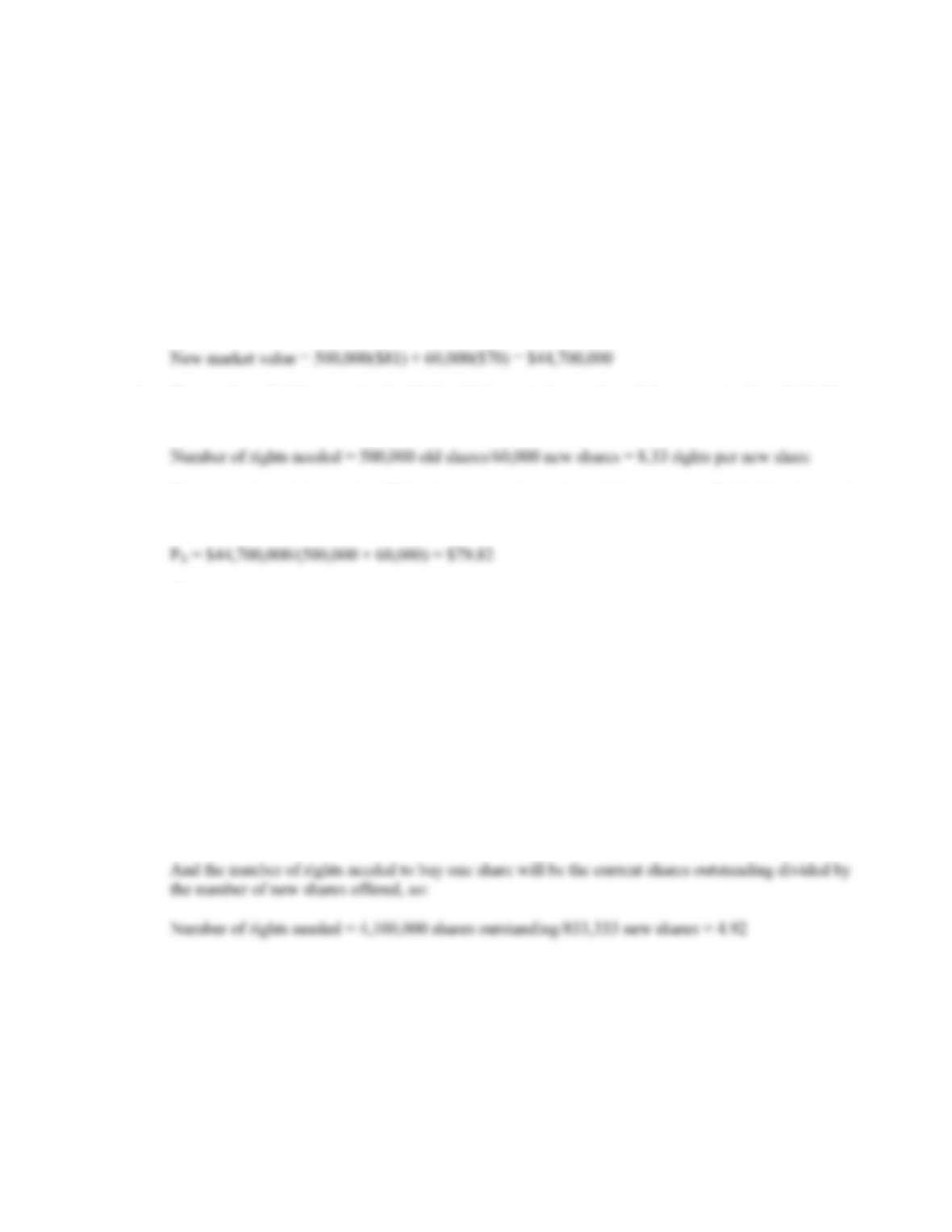B-268 SOLUTIONS
Solutions to Questions and Problems
NOTE: All end of chapter problems were solved using a spreadsheet. Many problems require multiple steps.
Due to space and readability constraints, when these intermediate steps are included in this solutions
manual, rounding may appear to have occurred. However, the final answer for each problem is found
without rounding during any step in the problem.
Basic
1. a. The new market value will be the current shares outstanding times the stock price plus the rights
offered times the rights price, so:
b. The number of rights associated with the old shares is the number of shares outstanding divided by
the rights offered, so:
c. The new price of the stock will be the new market value of the company divided by the total
number of shares outstanding after the rights offer, which will be:
d. The value of the right
Value of a right = \$81.00 – 79.82 = \$1.18
e. A rights offering usually costs less, it protects the proportionate interests of existing share-holders
and also protects against underpricing.
2. a. The maximum subscription price is the current stock price, or \$53. The minimum price is anything
greater than \$0.
b. The number of new shares will be the amount raised divided by the subscription price, so:
Number of new shares = \$40,000,000/\$48 = 833,333 shares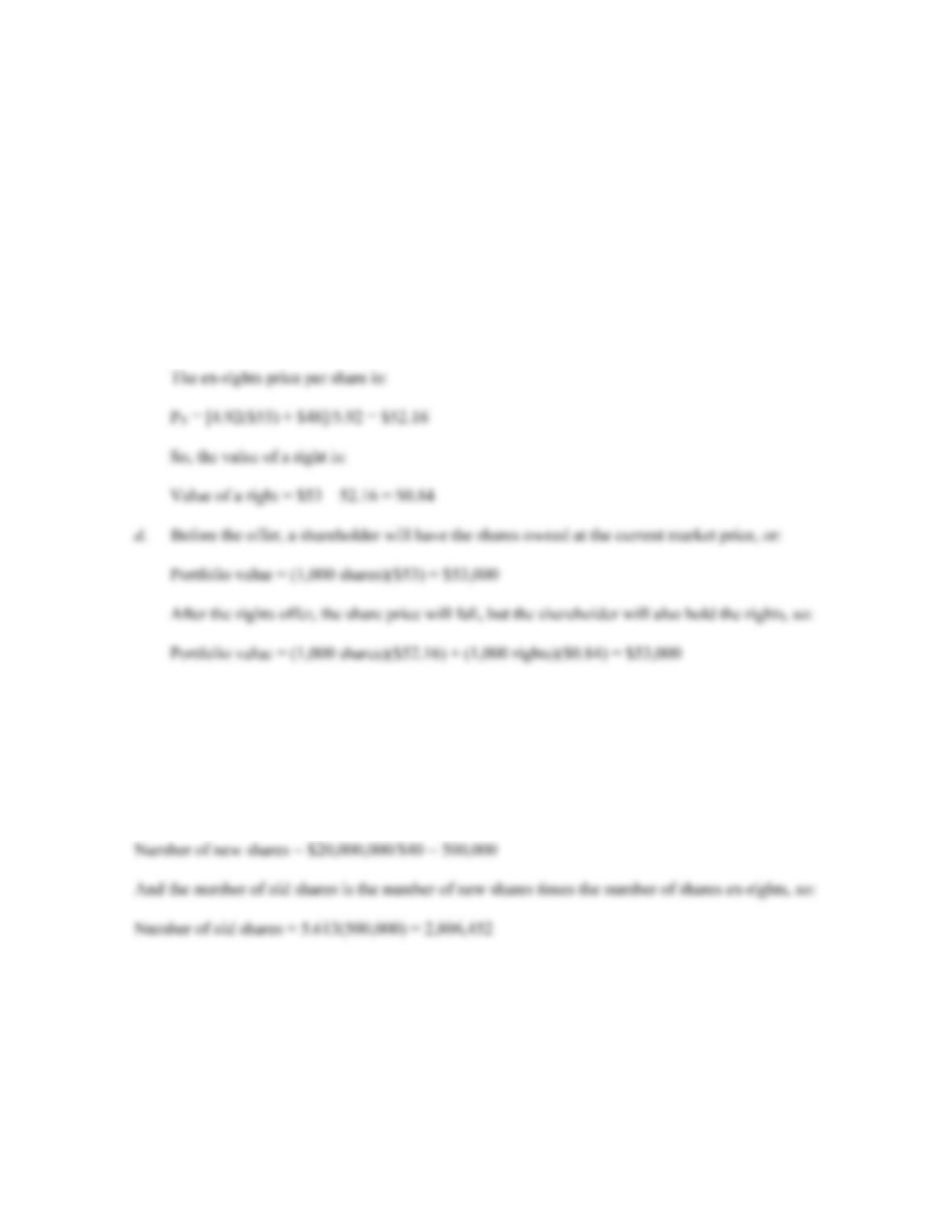CHAPTER 15 B-269
c. A shareholder can buy 4.92 rights on shares for:
4.92(\$53) = \$260.76
The shareholder can exercise these rights for \$48, at a total cost of:
\$260.76 + 48 = \$308.76
The investor will then have:
Ex-rights shares = 1 + 4.92
Ex-rights shares = 5.92
3. Using the equation we derived in Problem 2, part c to calculate the price of the stock ex-rights, we can
find the number of shares a shareholder will have ex-rights, which is:
P
X = \$74.80 = [N(\$81) + \$40]/(N + 1)
N = 5.613
The number of new shares is the amount raised divided by the per-share subscription price, so: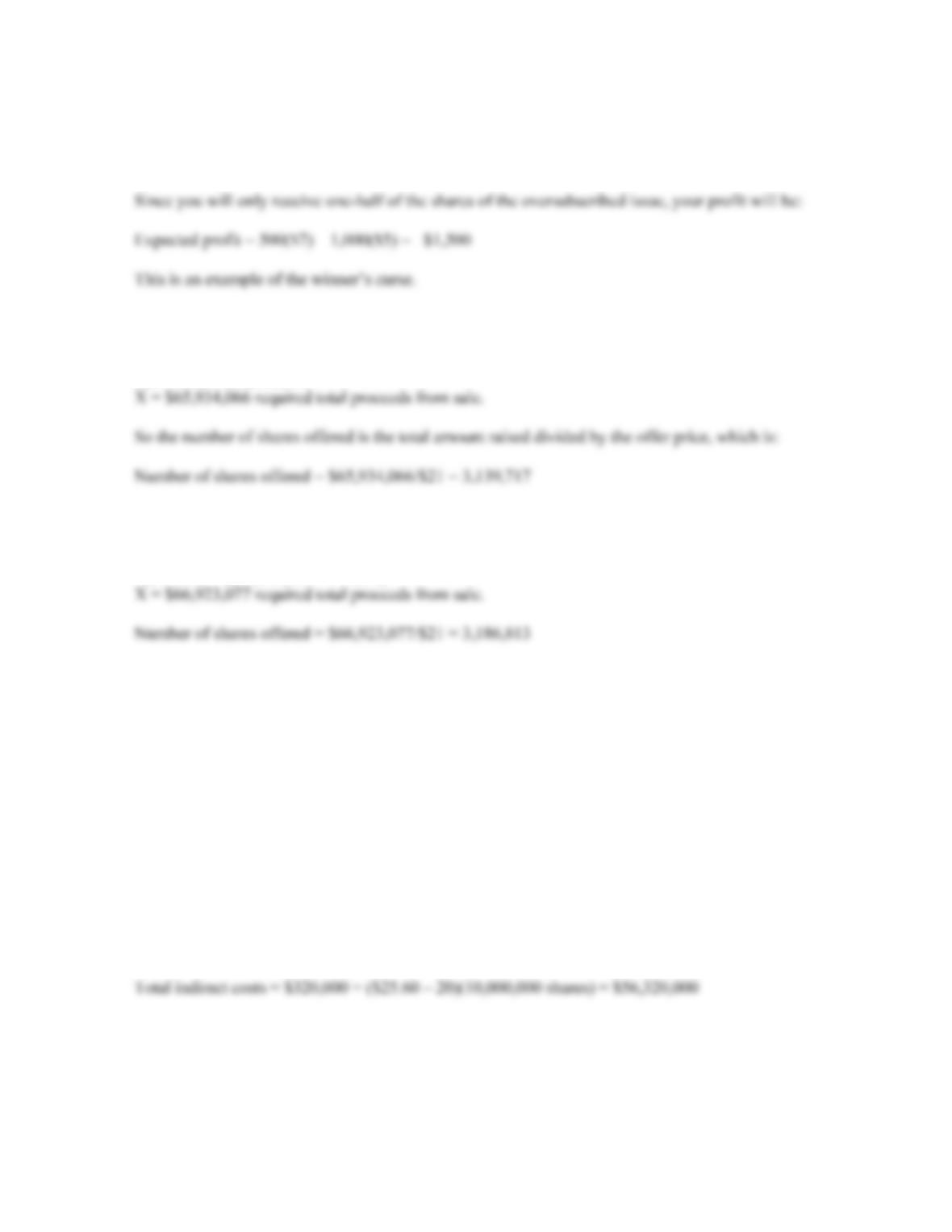B-270 SOLUTIONS
4. If you receive 1,000 shares of each, the profit is:
Profit = 1,000(\$7) – 1,000(\$5) = \$2,000
5. Using X to stand for the required sale proceeds, the equation to calculate the total sale proceeds,
including floatation costs is:
X(1 – .09) = \$60,000,000
6. This is basically the same as the previous problem, except we need to include the \$900,000 of expenses
in the amount the company needs to raise, so:
X(1 – .09) = (\$60,000,000 + 900,00)
7. We need to calculate the net amount raised and the costs associated with the offer. The net amount
raised is the number of shares offered times the price received by the company, minus the costs
associated with the offer, so:
Net amount raised = (10,000,000 shares)(\$18.20) – 900,000 – 320,000 = \$180,780,000
The company received \$180,780,000 from the stock offering. Now we can calculate the direct costs.
Part of the direct costs are given in the problem, but the company also had to pay the underwriters. The
stock was offered at \$20 per share, and the company received \$18.20 per share. The difference, which is
the underwriters spread, is also a direct cost. The total direct costs were:
Total direct costs = \$900,000 + (\$20 – 18.20)(10,000,000 shares) = \$18,900,000
We are given part of the indirect costs in the problem. Another indirect cost is the immediate price
appreciation. The total indirect costs were: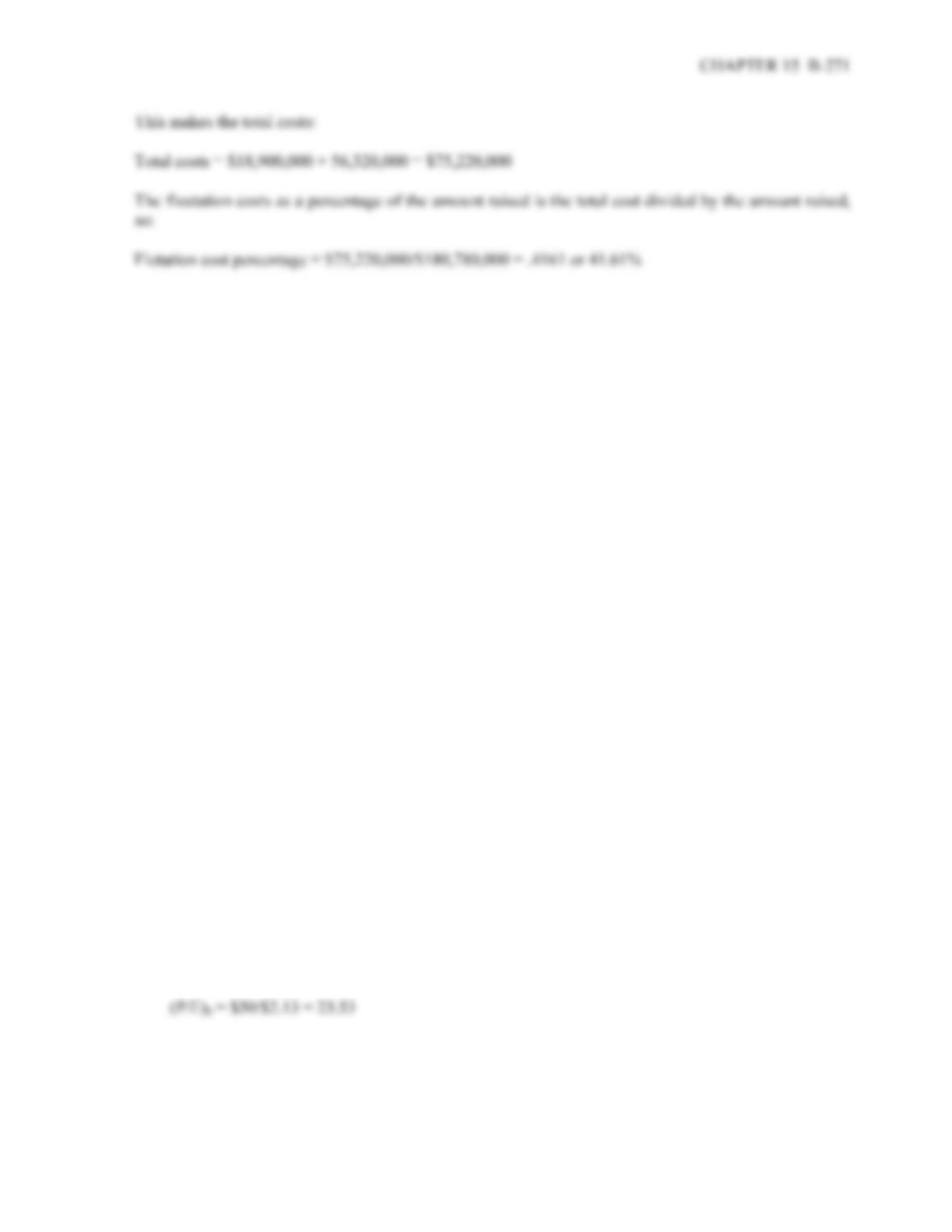8. The number of rights needed per new share is:
Number of rights needed = 120,000 old shares/25,000 new shares = 4.8 rights per new share.
Using PRO as the rights-on price, and PS as the subscription price, we can express the price per share of
the stock ex-rights as:
P
X = [NPRO + PS]/(N + 1)
a. P
X = [4.8(\$94) + \$94]/(4.80 + 1) = \$94.00; No change.
b. PX = [4.8(\$94) + \$90]/(4.80 + 1) = \$93.31; Price drops by \$0.69 per share.
c. PX = [4.8(\$94) + \$85]/(4.80 + 1) = \$92.45; Price drops by \$1.55 per share.
Intermediate
9. a. The number of shares outstanding after the stock offer will be the current shares outstanding, plus
the amount raised divided by the current stock price, assuming the stock price doesn’t change. So:
Number of shares after offering = 8,000,000 + \$35,000,000/\$50 = 8,700,000
Since the book value per share is \$18, the old book value of the shares is the current number of
shares outstanding times 18. From the previous solution, we can see the company will sell 700,000
shares, and these will have a book value of \$50 per share. The sum of these two values will give us
the total book value of the company. If we divide this by the new number of shares outstanding.
Doing so, we find the new book value per share will be:
New book value per share = [8,000,000(\$18) + 700,000(\$50)]/8,700,000 = \$23.53
The current EPS for the company is:
EPS0 = NI0/Shares0 = \$17,000,000/8,000,000 shares = \$2.13 per share
And the current P/E is: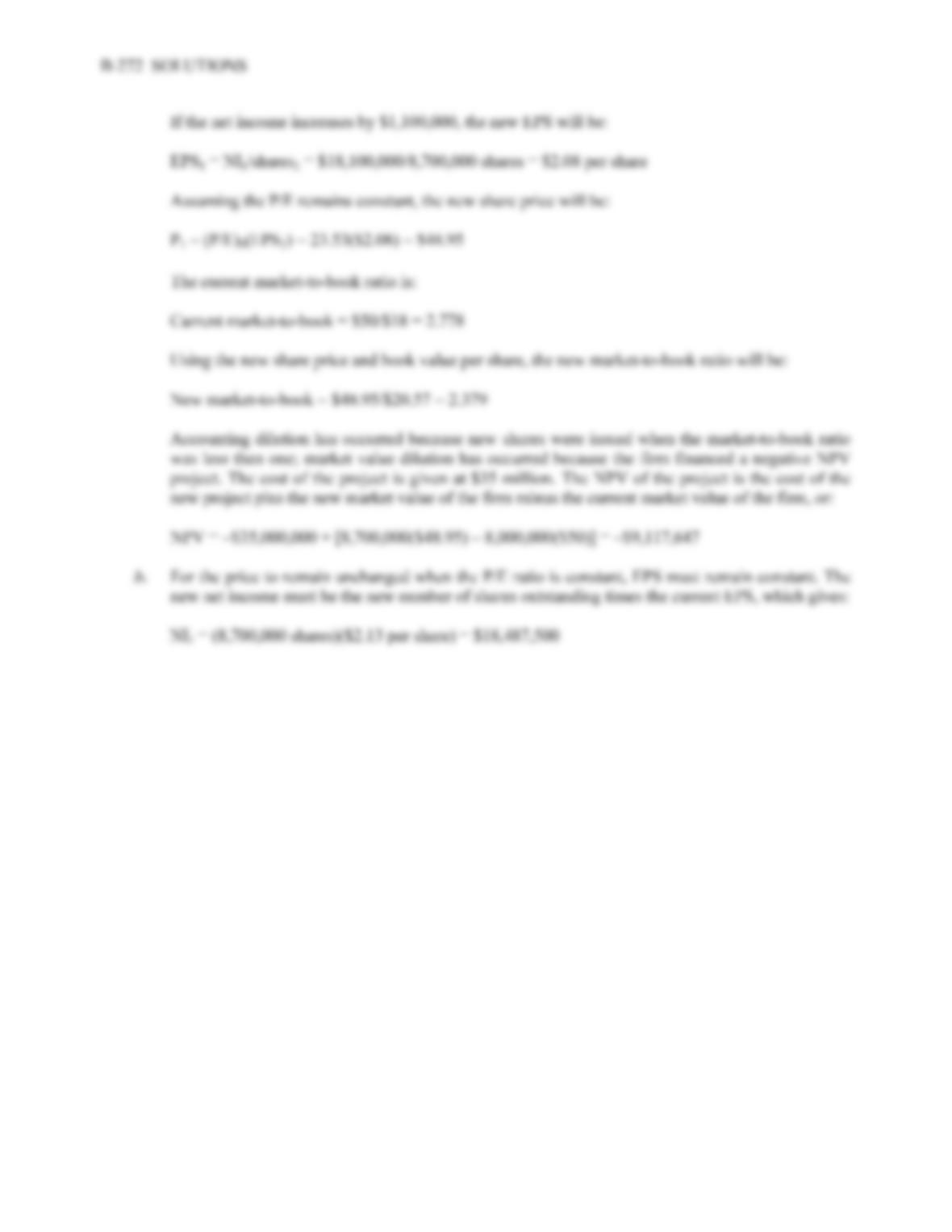10. The total equity of the company is total assets minus total liabilities, or:
Equity = \$8,000,000 – 3,400,000
Equity = \$4,600,000
So, the current ROE of the company is:
ROE0 = NI0/TE0 = \$900,000/\$4,600,000 = .1957 or 19.57%
The new net income will be the ROE times the new total equity, or:
NI1 = (ROE0)(TE1) = .1957(\$4,600,000 + 850,000) = \$1,066,304
The company’s current earnings per share are:
EPS0 = NI0/Shares outstanding0 = \$900,000/30,000 shares = \$30.00
The number of shares the company will offer is the cost of the investment divided by the current share
price, so:
Number of new shares = \$850,000/\$84 = 10,119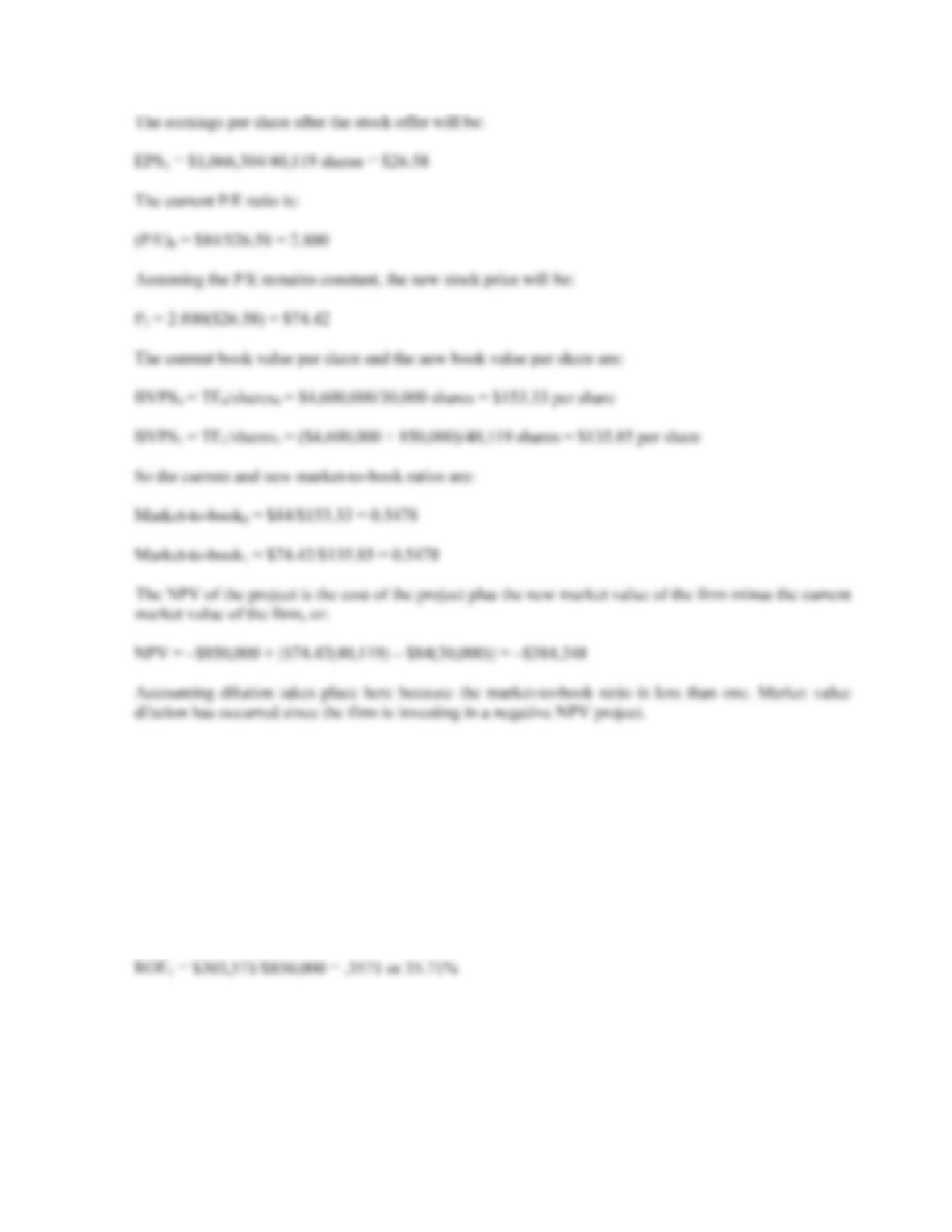CHAPTER 15 B-273
11. Using the P/E ratio to find the necessary EPS after the stock issue, we get:
P
1 = \$84 = 2.800(EPS1)
EPS1 = \$30.00
The additional net income level must be the EPS times the new shares outstanding, so:
NI = \$30(10,119 shares) = \$303,571
And the new ROE is: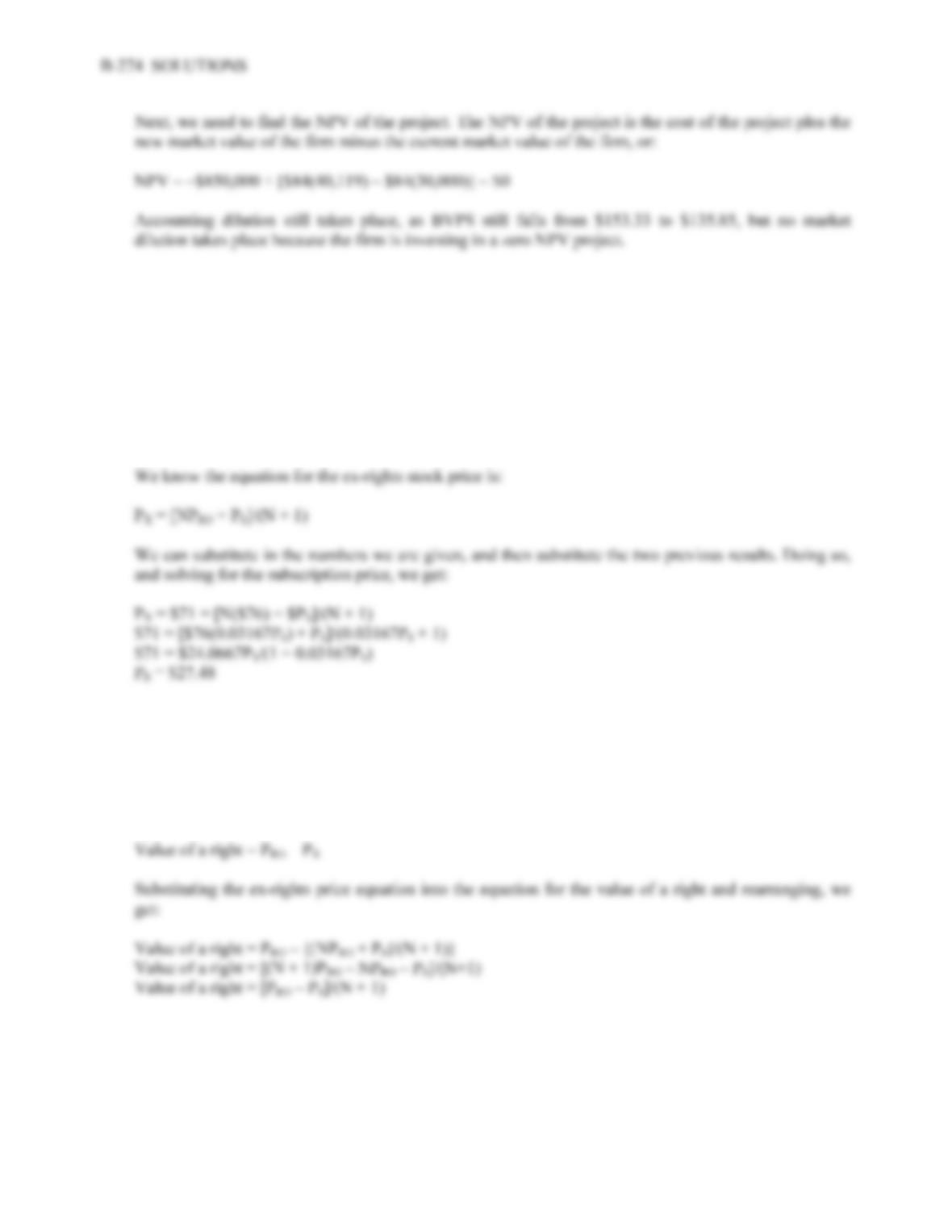12. The number of new shares is the amount raised divided by the subscription price, so:
Number of new shares = \$60,000,000/\$PS
And the ex-rights number of shares (N) is equal to:
N = Old shares outstanding/New shares outstanding
N = 19,000,000/(\$60,000,000/\$PS)
N = 0.03167PS
13. Using PRO as the rights-on price, and PS as the subscription price, we can express the price per share of
the stock ex-rights as:
P
X = [NPRO + PS]/(N + 1)
And the equation for the value of a right is: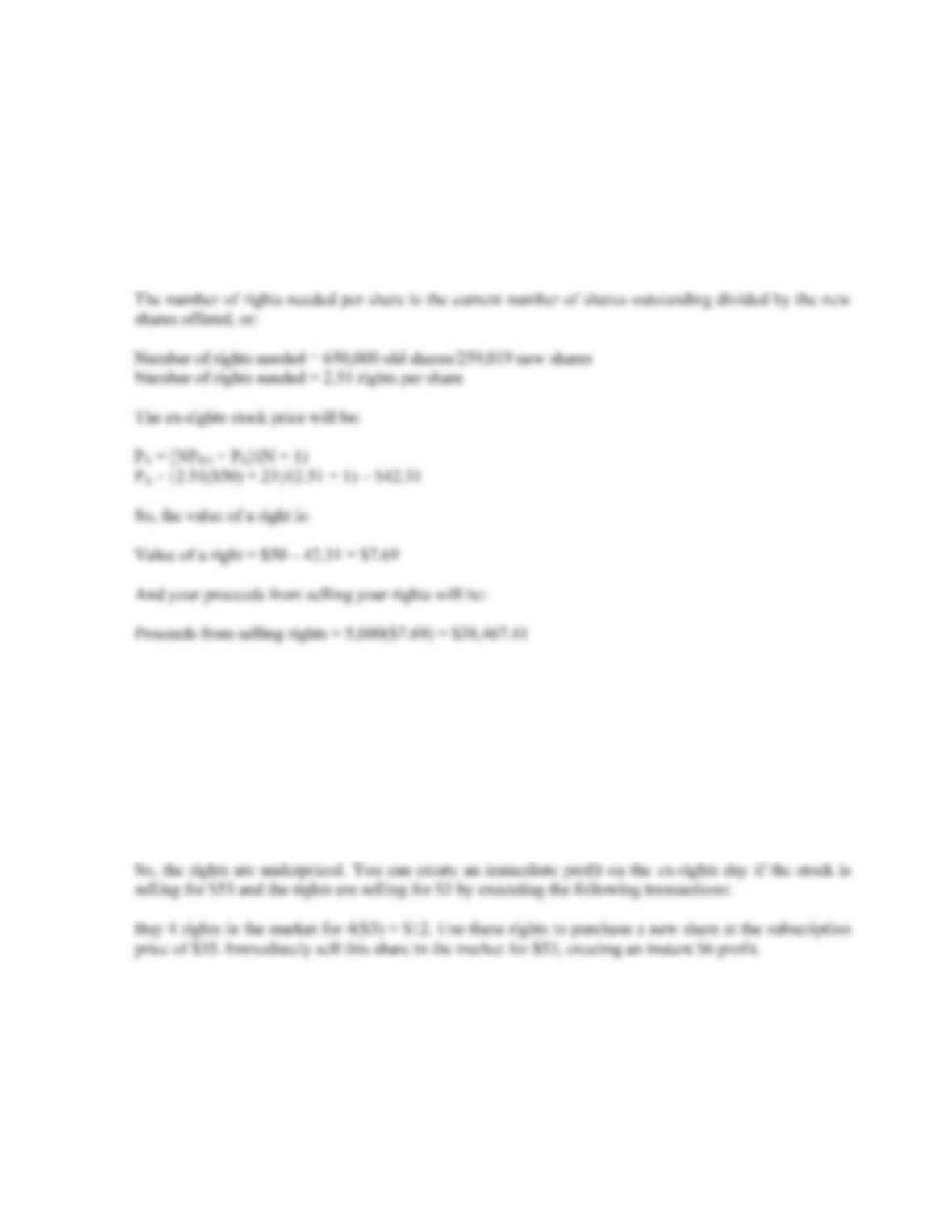CHAPTER 15 B-275
14. The net proceeds to the company on a per share basis is the subscription price times one minus the
Net proceeds to the company = \$23(1 – .06) = \$21.62 per share
So, to raise the required funds, the company must sell:
New shares offered = \$5,600,000/\$21.62 = 259,019
15. Using the equation for valuing a stock ex-rights, we find:
P
X = [NPRO + PS]/(N + 1)
P
X = [4(\$60) + \$35]/(4 + 1) = \$55
The stock is correctly priced. Calculating the value of a right, we find:
Value of a right = PRO – PX
Value of a right = \$60 – 53 = \$7

## Trusted by Thousands ofStudents

Here are what students say about us.